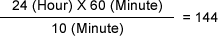Sound Level Meter FAQWhat is the maximum time for storage data to the SD card?The maximum setting time of the Measurement Time and Total Time are 199 hours 59 minutes 59 seconds. The minimum setting time of the Measurement Time is 0.1 second, and Total Time is 1 second. Memory mode has a function which enables storage of the measurement data automatically and repeatedly.

For example, set Measurement Time as 10 minutes and Total Time as 24 hours for measuring LAeq data. To execute the data storage in this setting, LAeq calculated data of the 10 minutes measurement is stored repeatedly to the memory for 24 hours. The number of the data can be obtained as follows;144 data are stored as one file.

When the memory mode is set as "1TWAuto", one data records each unit of LP/Leq/LE/LMX/LMN/Lpk and the number of data bytes is 86.

The number of bytes of one file is calculated as 144 x 86 = 12384 bytes. 367 bites data is added for management data, so 12751 bytes (approx. 13 k bytes) of memory are used in total.

When set the Total Time as 199 hours 59 minutes 59 seconds (719999 seconds) and Measurement Time as one second, the number of bytes is 719999 x 86 + 367 = 61920281 bytes (approx. 60 M bytes).

Furthermore, when the Total Time is set as 0, the measurement is stopped after file storage of single measurement data.

The principle of above function will be the same when the LA-0551 and LA-0552 real time analysis function is used.

For the LA-0554 sound recording function, please refer to another FAQ.

Revised:2007.09.18• Copyright © ONO SOKKI CO.,LTD All Rights Reserved.